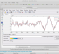## Noise estimation and thresholding methods in Discrete Wavelet TransformStarted by 2 years ago2 replieslatest reply 2 years ago104 views

I am a bit confused regarding the thresholding methods and noise estimation options found in Matlab regarding Discrete Wavelet Transform.

Please correct me if I am wrong.

I am understanding that noise estimation options are estimating the noise per scale (level dependent) or estimating the noise per the first level (level independent). Reading about thresholding methods, SURE or Bayes; for example, we have the option to estimate noise level dependent and level independent with both these methods. But both of these methods are considered adaptive or subband. So would this adaptability be only true if the level dependent noise estimation is chosen otherwise it will act as a fixed threshold but uses its relevant method of calculating the appropriate threshold?

Thank you

[ - ]Hİ,

Even if it is fixed, you apply in on sub-bands (there could be coefficients exceeding threshold or not). Coefficients are found for each level of tree, hence adaptive or sub-band processing becomes again.

[ - ]